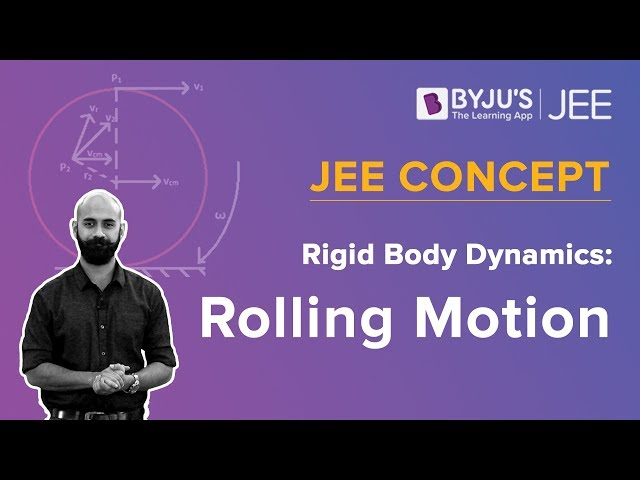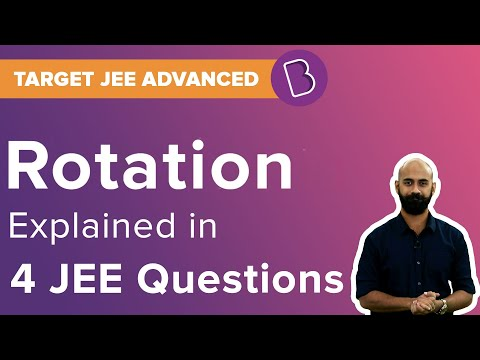# System Of Particles And Rotational Motion Class 11 Notes

## What is Translational Motion?

Let us understand translational motion with the help of examples. Let’s imagine a rectangular block placed on the slanting edge of a right-angled triangle. If the block is assumed to slide down this edge without any side movement, every point in the rectangular block experiences the same displacement and more importantly, the distance between the points is also maintained.

• In a pure translational motion, every point in the body experiences the same velocity be it at any instant of time. Both the points, P1 and P2 undergo the exact same motions. A car moving in a straight line, the path of a bullet out of a gun etc are examples of translational motion.

### What is Rotational Motion?

Lets us understand this by an example. Now let us imagine a circular block going down the edge of the right-angled triangle. Examining the location and orientation of different points on the cylindrical block will tell us something new. The points on the cylindrical body experience something much different than the rectangular block. As shown by the arrows in the diagram representing the velocity, each point experiences a different magnitude of velocity in a different direction. Here the points are arranged with respect to an axis of rotation.

• Rotation is what you achieve when you constrain a body and fix it along with a straight line. This means that the body can only turn around the line, which is defined as rotational motion. A ceiling fan, a potter’s wheel, a vehicle’s wheel are all examples of rotational motion.#### Watch The Below Videos to Know More:### Important Questions

Give the location of the center of mass of a

• (i) sphere,
• (ii) cylinder,
• (iii) ring, and
• (iv) cube, each of uniform mass density.

Does the centre of mass of a body necessarily lie inside the body?

1. Two particles, each of mass m and speed v, travel in opposite directions along parallel lines separated by a distance d. Show that the angular momentum vector of the two-particle system is the same whatever be the point about which the angular momentum is taken.
1. Torques of equal magnitude are applied to a hollow cylinder and a solid sphere, both having the same mass and radius. The cylinder is free to rotate about its standard axis of symmetry and the sphere is free to rotate about an axis passing through its centre. Which of the two will acquire a greater angular speed after a given time?

Students can find details about the system of particles and rotational motion class 11 notes from this article.

## Frequently asked Questions on CBSE Class 11 Physics Notes Chapter 7: System of Particles and Rotational Motion

### What are some of the examples of ‘torque’ in real life?

Opening a bottle cap or turning a steering wheel are examples of torque.

### What is meant by ‘Centre of mass’?

The center of mass is the unique point at the center of a distribution of mass in space that has the property that the weighted position vectors relative to this point sum to zero.

### What is meant by ‘Magnitude’?

Magnitude can be described as ‘distance or quantity’. It shows the direction or size that is absolute or relative in which an object moves in the sense of motion.